Search
Document Center

# Use ALS to predict ratings of songs

Last Updated: May 14, 2020

Use ALS to predict ratings of songs

Many people will visit a movie recommendation website to check the rating of a movie before they watch it. After they watch a movie, they will also assign a rating to the movie. Everyone has a rating system in their mind. The rating of a commodity, song, or movie reflects whether the user likes or dislikes it. If a content provider can estimate the ratings to be assigned by its users, it can understand its users in a better way and then make more precise recommendations. This topic describes how to use Alternating Least Square (ALS), a factorization algorithm, to predict the ratings of a song or movie assigned by users.

## ALS introduction

ALS is a model-based recommendation algorithm. It factorizes models through sparse matrix factorization, and predicts the values of missing entries. In this way, a basic model is trained. The model is then used to make predictions based on new user and item data. ALS uses the alternating least squares method to calculate missing entries. The alternating least squares method is developed based on the least squares method.

ALS is a type of user-item based collaborative filtering, also known as hybrid collaborative filtering.

In this topic, we use music rating as an example to introduce how ALS works. The source dataset, Matrix A, contains the ratings of songs assigned by all listeners. The ratings may be sparse because not every listener has listened to all the songs in the library and not all the songs are rated by every listener.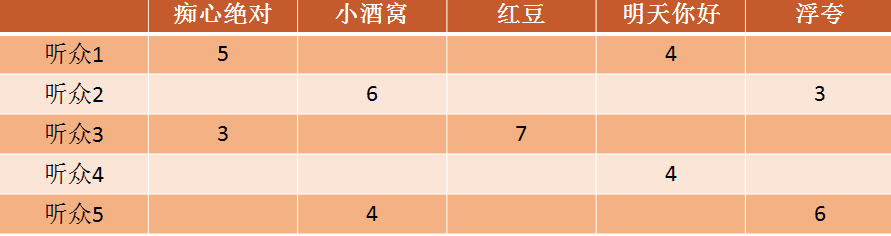ALS factorizes Matrix A to the product of the transposes of Matrix X and Matrix Y.

``Matrix A = Transpose of Matrix X × Transpose of Matrix Y``

The columns in Matrix X and rows in Matrix Y are known as factors in ALS. These factors have implicit definitions. Matrix X and Matrix Y contain three factors: personality, education level, and interests. Matrix X and Matrix Y factorized from Matrix A are expressed as follows.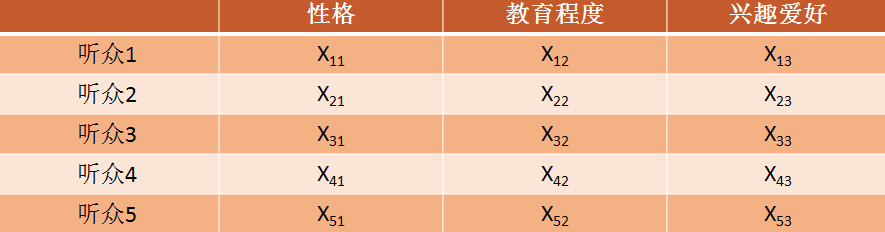(Matrix X)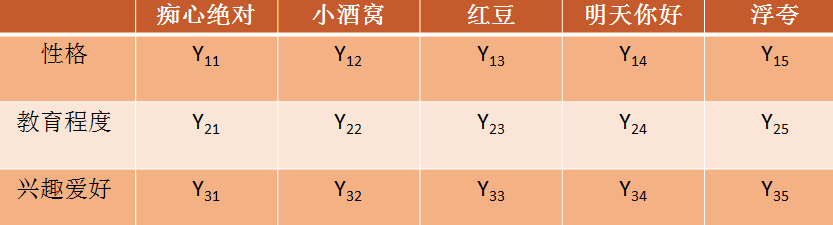(Matrix Y)

Based on the factorized data, rating predictions can be easily made. For example, Listener 6 has never listened to the song Red Bean but we have obtained the Vector M of Listener 6 from Matrix X. To predict the rating of Red Bean by Listener 6, we only need to multiply Vector M of Listener 6 by Vector M of Red Bean in Matrix Y.

## Use ALS in Alibaba Cloud Machine Learning Platform for AI (PAI)

Now we create an experiment in Alibaba Cloud PAI based on the preceding ALS use case. The experiment consists of the input data and ALS components. You can find the template of this use case on the Home page of PAI Studio.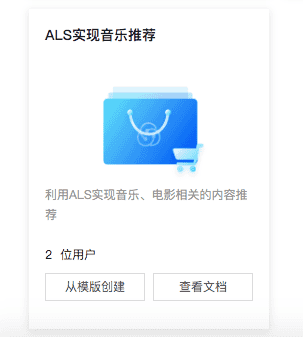The following figure shows the created experiment.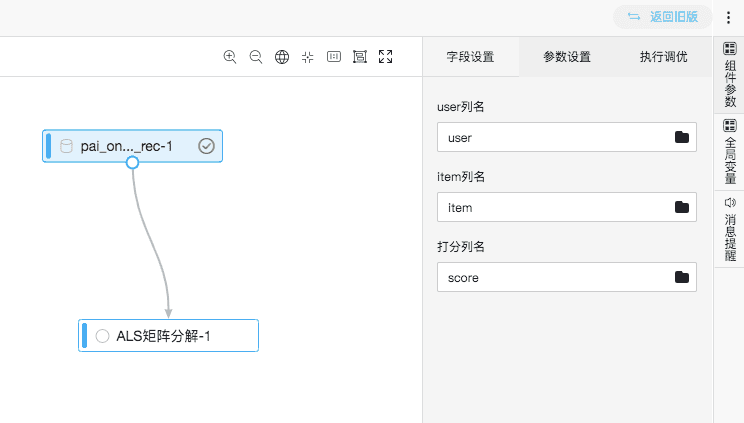### 1. Data source

The input data contains the following fields.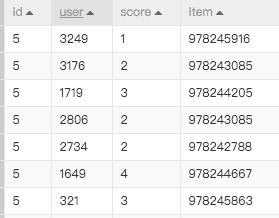• user: user ID.
• item: song ID.
• score: the rating of the song assigned by the relevant user.

### 2. ALS matrix factorization

You must specify the fields as shown in the following figure.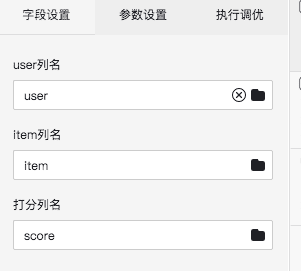Parameter Description Valid value Required or not and default value
userColName The name of the user column. The column type must be bigint. The entries do not need to be continuously numbered. Required.
itemColName The name of the item column. The column type must be bigint. The entries do not need to be continuously numbered. Required.
rateColName The name of the score column. The column type must be numeric. Required.
numFactors The number of factors. Positive integer. Optional. Default value: 100.
numIter The number of iterations. Positive integer. Optional. Default value: 10.
lambda Regularization coefficient. Floating point. Optional. Default value: 0.1.
implicitPref Specifies whether the implicit preference model is used. Boolean. Optional. Default value: false.
alpha Implicit preference coefficient. Floating point larger than 0. Optional. Default value: 40.

### 3. Prediction result analysis

In this experiment, two tables are output, which correspond to Matrix X and Matrix Y described in the ALS introduction.

The Matrix X table is as follows.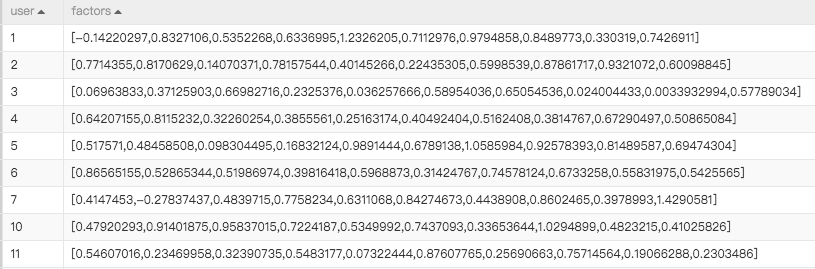The Matrix Y table is as follows.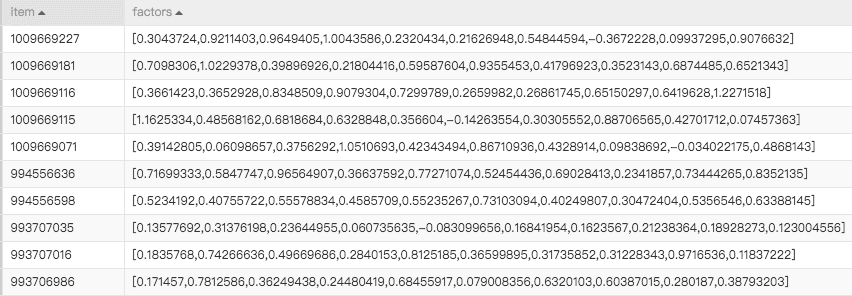To predict the rating of item 994556636 made by user1, you only need to multiply the following vectors together.

• User1: [-0.14220297,0.8327106,0.5352268,0.6336995,1.2326205,0.7112976,0.9794858,0.8489773,0.330319,0.7426911]
• item994556636: [0.71699333,0.5847747,0.96564907,0.36637592,0.77271074,0.52454436,0.69028413,0.2341857,0.73444265,0.8352135]